Next: Boundary conditions for and Up: Dielectric and magnetic media Previous: Introduction

## Polarization

The terrestrial environment is characterized by dielectric media (e.g., air, water) which are, for the most part, electrically neutral, since they are made up of neutral atoms and molecules. However, if these atoms and molecules are placed in an electric field then they tend to polarize. Suppose that when a given neutral molecule is placed in an electric field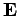, the centre of charge of its constituent electrons (whose total charge is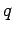) is displaced by a distance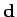with respect to the centre of charge of its constituent atomic nucleus. The dipole moment of the molecule is defined. If there aresuch molecules per unit volume then the electric polarization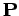(i.e., the dipole moment per unit volume) is given by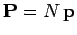. More generally,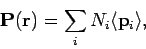(803)

whereis the average dipole moment of the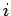th type of molecule in the vicinity of point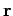, and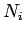is the average number of such molecules per unit volume at.

Consider an infinitesimal cube of dielectric material with-coordinates betweenand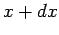,-coordinates betweenand, and-coordinates betweenand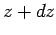. Suppose that the dielectric consists of electrically neutral polar molecules, of varying number density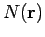, whose electrons, charge, displace a constant distancefrom the nuclei, charge. Thus, the dipole moment per unit volume is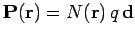. Due to the polarization of the molecules, a net chargeenters the bottom face of the cube, perpendicular to the-axis, whilst a net charge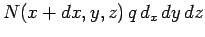leaves the top face. Hence, the net charge acquired by the cube due to molecular polarization in the-direction is. There are analogous contributions due to polarization in the- and-directions. Hence, the net charge acquired by the cube due to molecular polarization is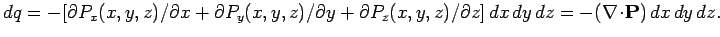Thus, it follows that the charge density acquired by the cube due to molecular polarization is simply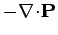.

As explained above, it is easily demonstrated that any divergence of the polarization field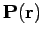of a dielectric medium gives rise to an effective charge density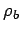in the medium, where(804)

This charge density is attributable to bound charges (i.e., charges which arise from the polarization of neutral atoms), and is usually distinguished from the charge density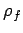due to free charges, which represents a net surplus or deficit of electrons in the medium. Thus, the total charge densityin the medium is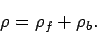(805)

It must be emphasized that both terms in this equation represent real physical charge. Nevertheless, it is useful to make the distinction between bound and free charges, especially when it comes to working out the energy associated with electric fields in dielectric media.

Gauss' law takes the differential form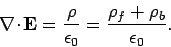(806)

This expression can be rearranged to give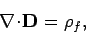(807)

where(808)

is termed the electric displacement, and has the same dimensions as(dipole moment per unit volume). Gauss' theorem tells us that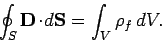(809)

In other words, the flux of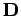out of some closed surfaceis equal to the total free charge enclosed within that surface. Unlike the electric field(which is the force acting on a unit charge), or the polarization(the dipole moment per unit volume), the electric displacementhas no clear physical meaning. The only reason for introducing this quantity is that it enables us to calculate electric fields in the presence of dielectric materials without first having to know the distribution of bound charges. However, this is only possible if we have a constitutive relation connectingand. It is conventional to assume that the induced polarizationis directly proportional to the electric field, so that(810)

where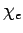is termed the electric susceptibility of the medium. It follows that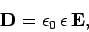(811)

where(812)

is termed the dielectric constant or relative permittivity of the medium. (Likewise,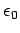is termed the permittivity of free space.) Note that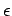is dimensionless. It follows from Eqs. (807) and (811) that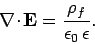(813)

Thus, the electric fields produced by free charges in a uniform dielectric medium are analogous to those produced by the same charges in a vacuum, except that they are reduced by a factor. This reduction can be understood in terms of a polarization of the atoms or molecules of the dielectric medium that produces electric fields in opposition to those generated by the free charges. One immediate consequence of this is that the capacitance of a capacitor is increased by a factorif the empty space between the electrodes is filled with a dielectric medium of dielectric constant(assuming that fringing fields can be neglected).

It must be understood that Eqs. (810)-(813) are just an approximation which is generally found to hold under terrestrial conditions (provided that the fields are not too large) for isotropic media. For anisotropic media (e.g., crystals), Eq. (811) generalizes to(814)

whereis a second-rank tensor known as the dielectric tensor. For strong electric fields,ceases to vary linearly with. Indeed, for sufficiently strong electric fields, neutral molecules are disrupted, and the whole concept of a dielectric medium becomes meaningless.Next: Boundary conditions for and Up: Dielectric and magnetic media Previous: Introduction
Richard Fitzpatrick 2006-02-02